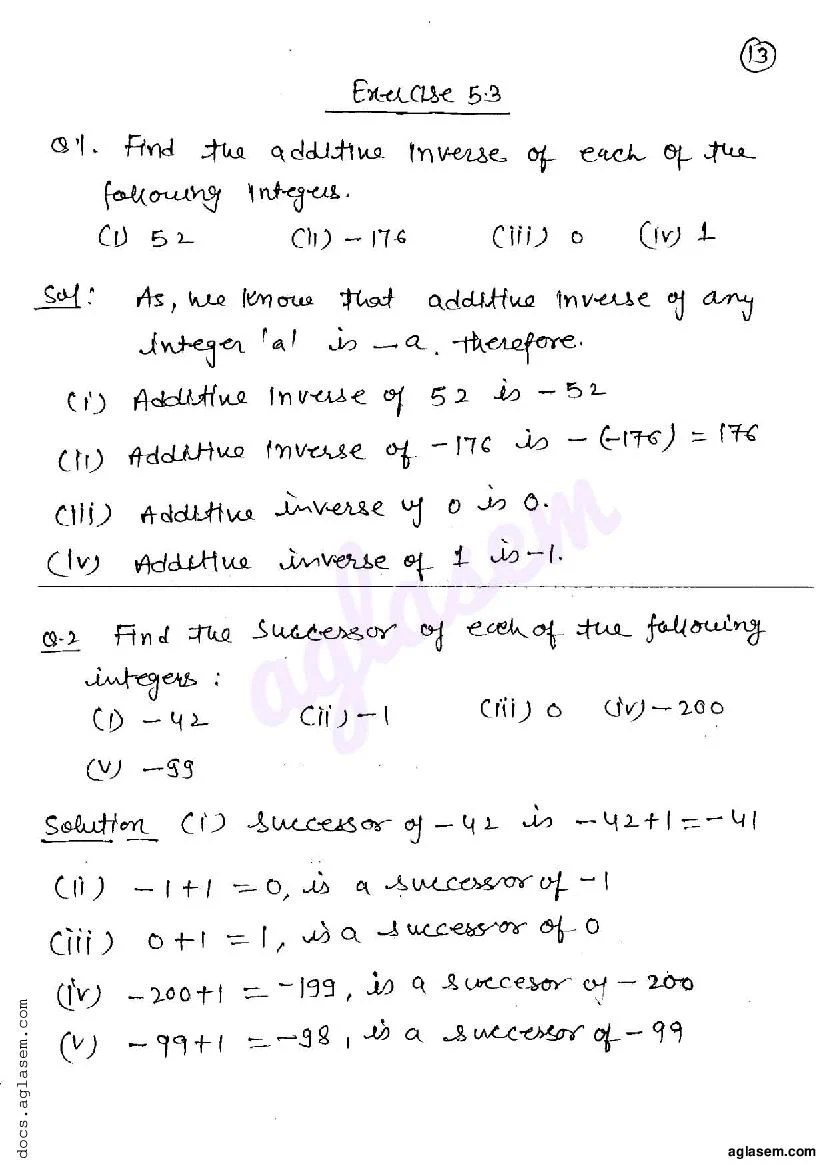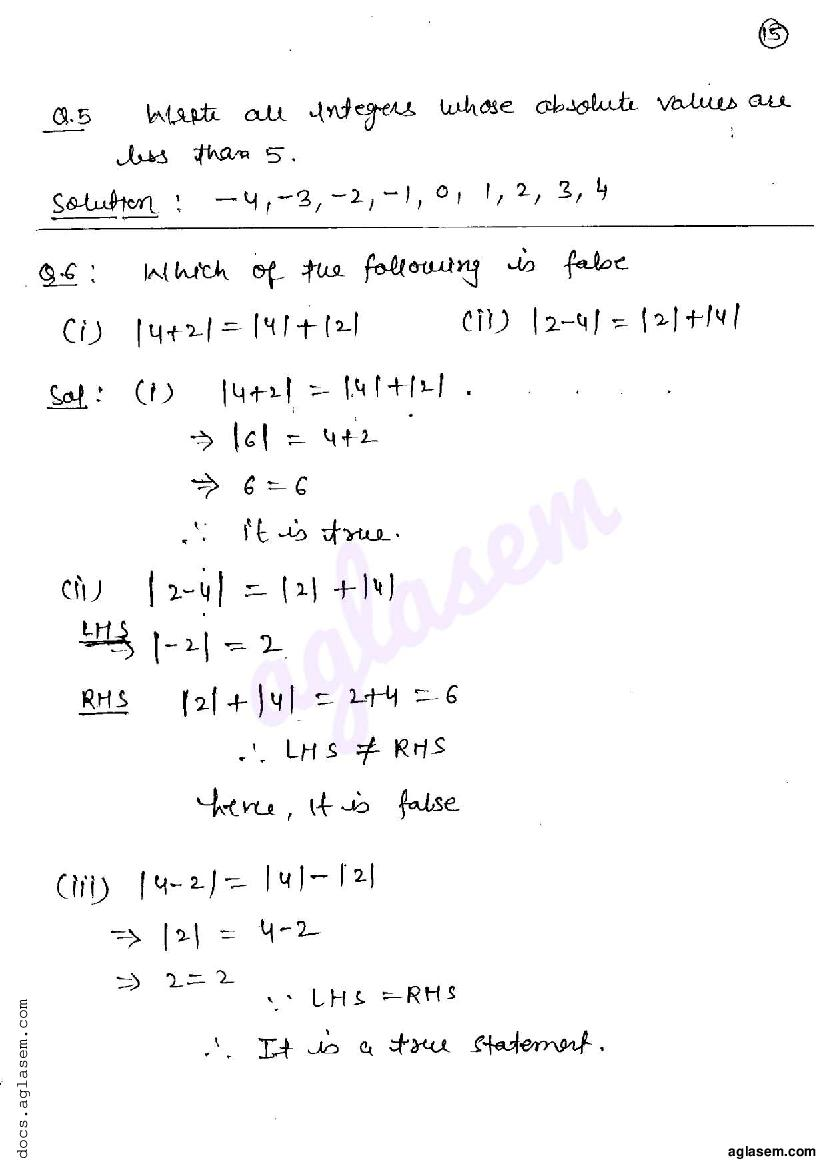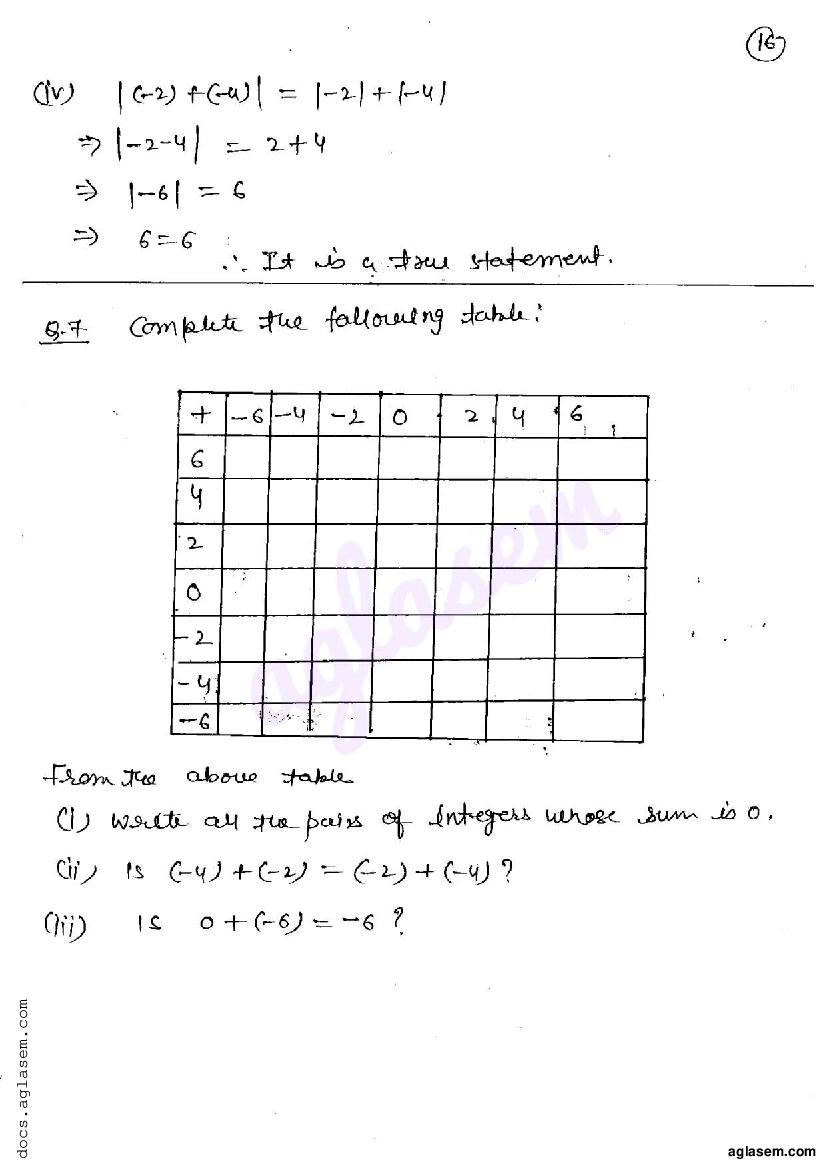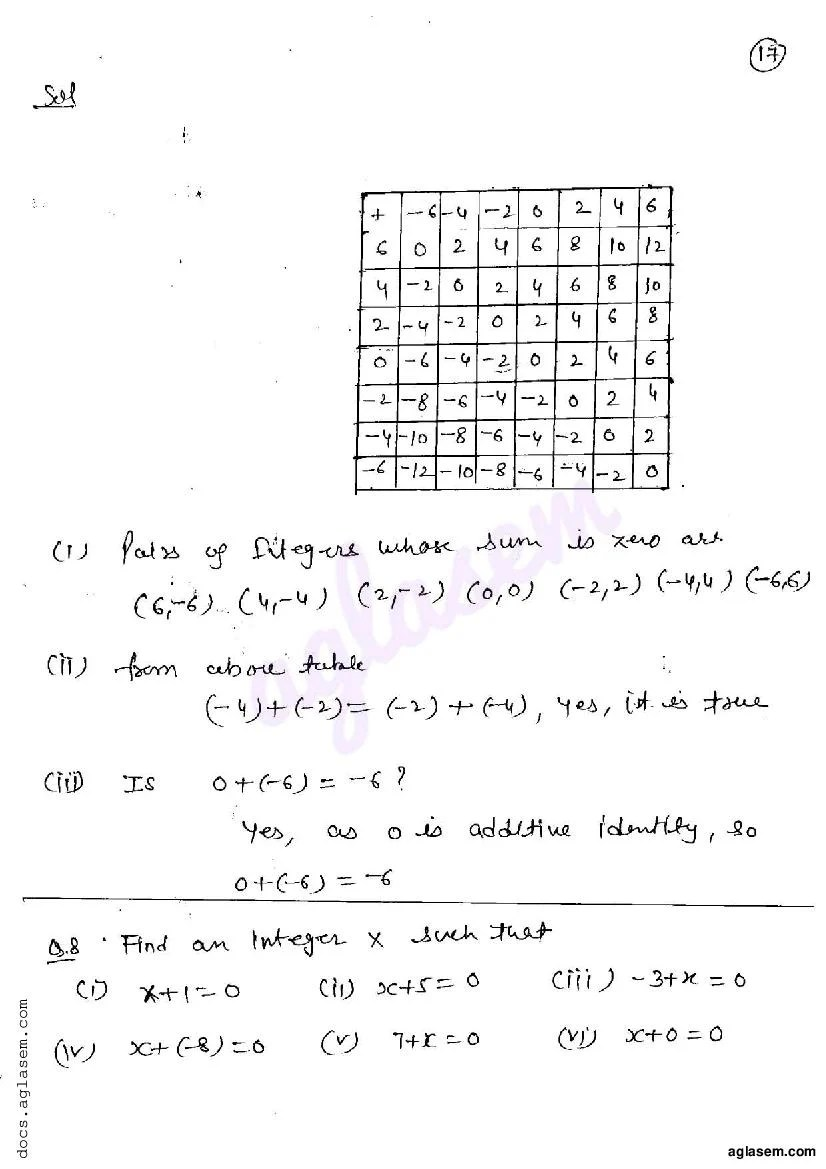# RD Sharma Solutions Class 6 Chapter 5 Negative Numbers and Integers Exercise 5.3

Here you can get free RD Sharma Solutions for Class 6 Maths Chapter 5 Negative Numbers and Integers Exercise 5.3. All RD Sharma Book Solutions are given here exercise wise for the chapter Negative Numbers and Integers. RD Sharma Solutions are helpful in the preparation of several school level, graduate and undergraduate level competitive exams. Practicing questions from RD Sharma Mathematics Solutions for Class 6 Chapter 5 Negative Numbers and Integers is proven to enhance your math skills.

 Class: Class 6th Chapter: Chapter 5 Exercise: Exercise 5.3 Name: Negative Numbers and Integers

## RD Sharma Solutions Class 6 Chapter 5 Negative Numbers and Integers Exercise 5.3

RD Sharma Class 6 Solutions Chapter 5 for Negative Numbers and Integers Exercise 5.3 are given below.

RD Sharma Solutions Class 6 Maths Chapter 5 Negative Numbers and Integers Exercise 5.3RD Sharma Solutions for Class 6 Maths PDF download for Chapter 5 Negative Numbers and Integers Exercise 5.3 is available here for free.

 « Previous Next »

### RD Sharma Solutions Class 6 Chapter 5 Exercise Wise

You can also see RD Sharma Solutions of all exercises of Chapter 5 – Negative Numbers and Integers from here.

### RD Sharma Solutions Class 6 Chapter Wise

If you want RD Sharma solutions of any topic other than Chapter 5 Negative Numbers and Integers, check it from here. Mathematics by RD Sharma has all chapters that are in your Class 6 syllabus.

Click to rate this post!
[Total: 1 Average: 5]